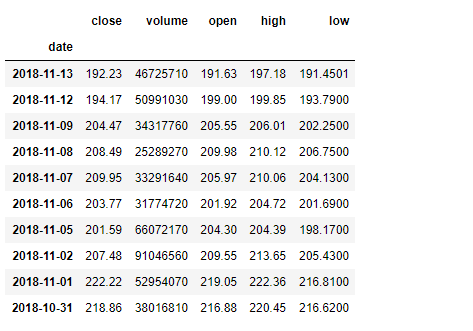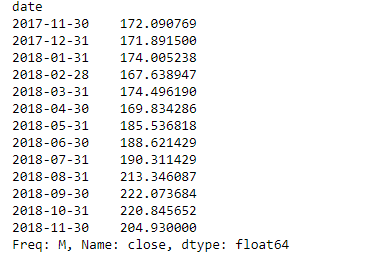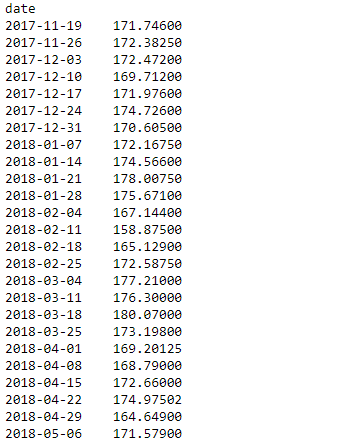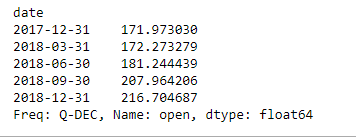# Python | Pandas dataframe.resample()

• Difficulty Level : Basic
• Last Updated : 22 Oct, 2019

Python is a great language for doing data analysis, primarily because of the fantastic ecosystem of data-centric python packages. Pandas is one of those packages and makes importing and analyzing data much easier.

Pandas` dataframe.resample()` function is primarily used for time series data.
A time series is a series of data points indexed (or listed or graphed) in time order. Most commonly, a time series is a sequence taken at successive equally spaced points in time. It is a Convenience method for frequency conversion and resampling of time series. Object must have a datetime-like index (DatetimeIndex, PeriodIndex, or TimedeltaIndex), or pass datetime-like values to the on or level keyword.

Syntax : DataFrame.resample(rule, how=None, axis=0, fill_method=None, closed=None, label=None, convention=’start’, kind=None, loffset=None, limit=None, base=0, on=None, level=None)

Parameters :
rule : the offset string or object representing target conversion
axis : int, optional, default 0
closed : {‘right’, ‘left’}
label : {‘right’, ‘left’}
convention : For PeriodIndex only, controls whether to use the start or end of rule
loffset : Adjust the resampled time labels
base : For frequencies that evenly subdivide 1 day, the “origin” of the aggregated intervals. For example, for ‘5min’ frequency, base could range from 0 through 4. Defaults to 0.
on : For a DataFrame, column to use instead of index for resampling. Column must be datetime-like.
level : For a MultiIndex, level (name or number) to use for resampling. Level must be datetime-like.

Resampling generates a unique sampling distribution on the basis of the actual data. We can apply various frequency to resample our time series data. This is a very important technique in the field of analytics.
Most commonly used time series frequency are –
W : weekly frequency
M : month end frequency
SM : semi-month end frequency (15th and end of month)
Q : quarter end frequency

There are many other types of time series frequency available. Let’s see how to apply these time series frequency on data and resample it.

This is a stock price data of Apple for a duration of 1 year from (13-11-17) to (13-11-18)

Example #1: Resampling the data on monthly frequency

 `# importing pandas as pd``import` `pandas as pd`` ` `# By default the "date" column was in string format,``# we need to convert it into date-time format`` ` `# parse_dates =["date"], converts the "date" ``# column to date-time format. We know that ``# resampling works with time-series data only``# so convert "date" column to index`` ` `# index_col ="date", makes "date" column, the index of the data frame``df ``=` `pd.read_csv(``"apple.csv"``, parse_dates ``=``[``"date"``], index_col ``=``"date"``)`` ` `# Printing the first 10 rows of dataframe``df[:``10``]``# Resampling the time series data based on months``# we apply it on stock close price``# 'M' indicates month``monthly_resampled_data ``=` `df.close.resample(``'M'``).mean()`` ` `# the above command will find the mean closing price``# of each month for a duration of 12 months.``monthly_resampled_data`

Output :Example #2: Resampling the data on weekly frequency

 `# importing pandas as pd``import` `pandas as pd`` ` `# We know that resampling works with time-series data``# only so convert "date" column to index``# index_col ="date", makes "date" column.`` ` `df ``=` `pd.read_csv(``"apple.csv"``, parse_dates ``=``[``"date"``], index_col ``=``"date"``)`` ` `# Resampling the time series data based on weekly frequency``# we apply it on stock open price 'W' indicates week``weekly_resampled_data ``=` `df.``open``.resample(``'W'``).mean()`` ` `# find the mean opening price of each week ``# for each week over a period of 1 year.``weekly_resampled_data`

Output :Example #3: Resampling the data on Quarterly frequency

 `# importing pandas as pd``import` `pandas as pd`` ` `# We know that resampling works with time-series``#  data only so convert our "date" column to index``# index_col ="date", makes "date" column``df ``=` `pd.read_csv(``"apple.csv"``, parse_dates ``=``[``"date"``], index_col ``=``"date"``)`` ` `# Resampling the time series data``#  based on Quarterly frequency``# 'Q' indicates quarter`` ` `Quarterly_resampled_data ``=` `df.``open``.resample(``'Q'``).mean()`` ` `# mean opening price of each quarter``# over a period of 1 year.``Quarterly_resampled_data`

Output :My Personal Notes arrow_drop_up